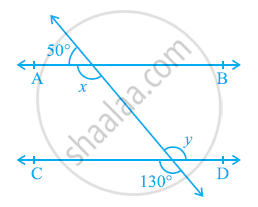# In the given figure, find the values of x and y and then show that AB || CD. - Mathematics

In the given figure, find the values of x and y and then show that AB || CD.#### Solution

It can be observed that,

50º + x = 180º (Linear pair)

x = 130º        … (1)

Also, y = 130º (Vertically opposite angles)

As x and y are alternate interior angles for lines AB and CD and also measures of these angles are equal to each other, therefore, line AB || CD.

Concept: Lines Parallel to the Same Line
Is there an error in this question or solution?
Chapter 6: Lines and Angles - Exercise 6.2 [Page 103]

#### APPEARS IN

NCERT Class 9 Maths
Chapter 6 Lines and Angles
Exercise 6.2 | Q 1 | Page 103

Share